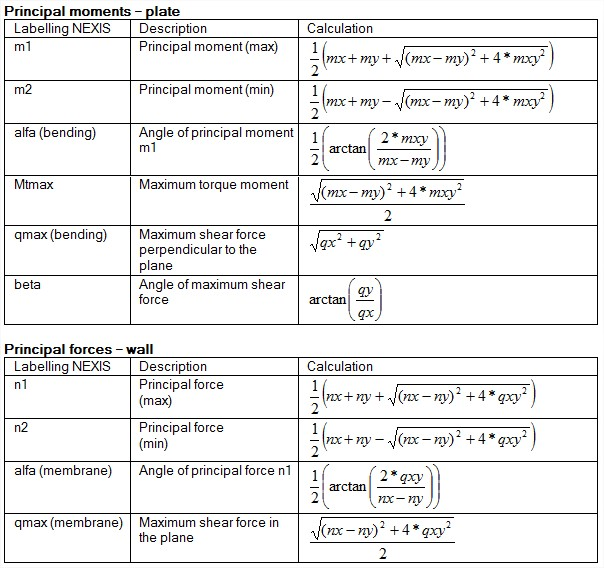# How are internal forces and stresses of 2D elements considered and calculated in SCIA Engineer?

Conventions for the results on 2D members:

### 1. Basic magnitudes = Characteristic values

Bending (plates, shells)

· *Bending moments mx, my

· *Torsion moment mxy

· *Shear forces qx, qy (=vx, vy)

Membrane effects (walls, shells)

· *Membrane forces nx, ny

· *Shear force qxy (=nxy)

### 2. Principal magnitudes

The principal magnitudes give the results according to the axes of the directions of the largest stresses (principal directions). These directions are defined with the help of the Mohr's circle.### 3. Dimensional magnitudes = Design values

To derive the dimensional magnitudes from the basic magnitudes, formulas from the Eurocode are used.